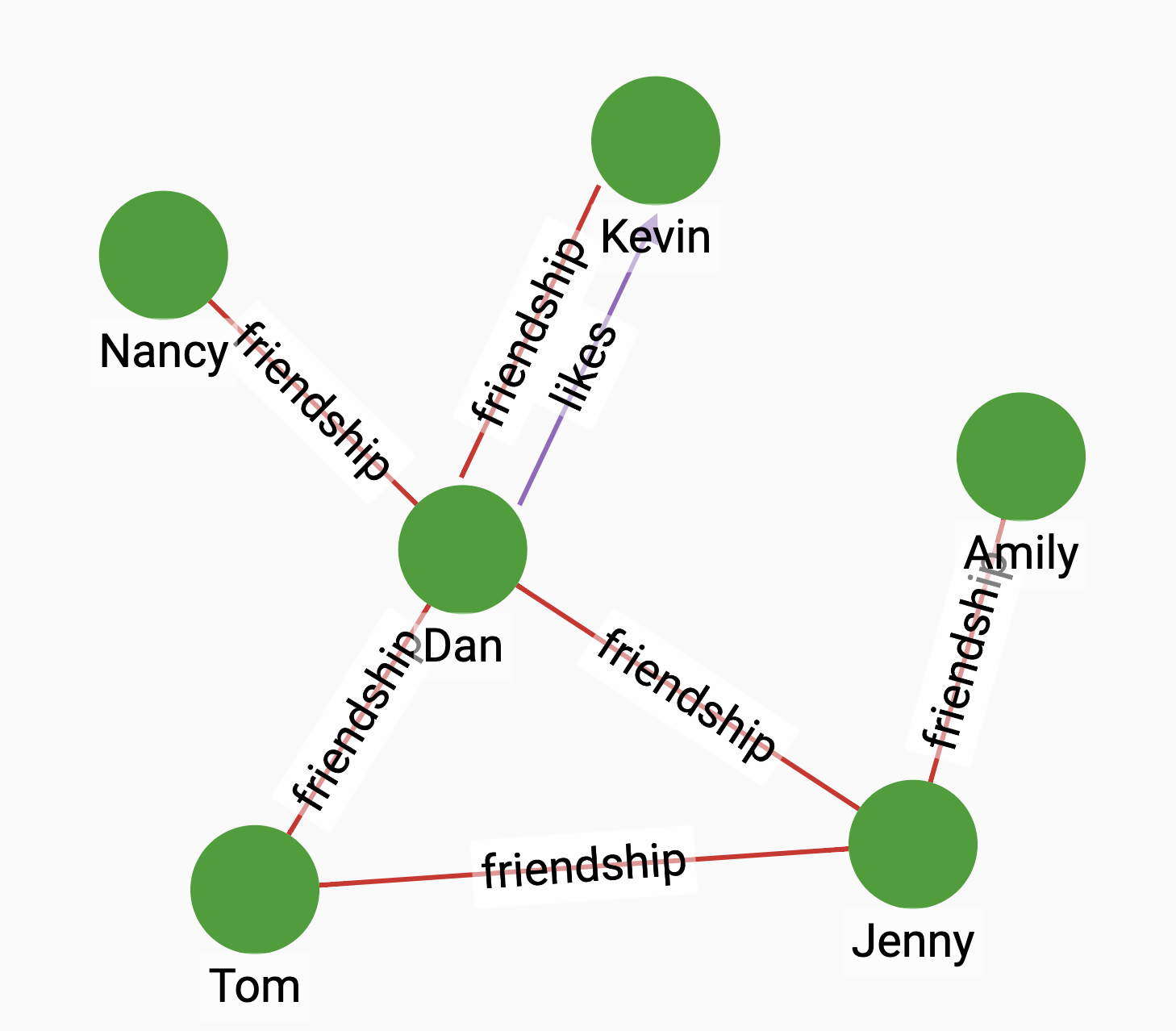# Preferential Attachment

Preferential Attachment is a measure to compute the closeness of vertices, based on the number of their neighbors. The algorithm returns the product two vertices' number of neighbors.

## Specification

``````CREATE QUERY tg_preferential_attachment(VERTEX a, VERTEX b,
SET<STRING> e_type)``````

### Time complexity

The algorithm has a time complexity of $O(D1 + D2)$, where D1 and D2 are the degrees of the two vertices.

## Parameters

Name Description Data type

`a`

A vertex.

`VERTEX`

`b`

A vertex.

`VERTEX`

`e_type`

Edge types to traverse.

`SET<STRING>`

## Return value

The product of the number of neighbors of the two vertices.

## Example

Suppose we have the following graph:Since Dan has four neighbors, while Jenny has three, the return value of the algorithm is $3*4=12$.

• Query

• Result

``RUN QUERY preferential_attachment (("Jenny", "person"), ("Dan", "person"), ["friendship"])``
``````{
"error": false,
"message": "",
"version": {
"schema": 1,
"edition": "enterprise",
"api": "v2"
},
"results": [{"closeness": 12}]
}``````